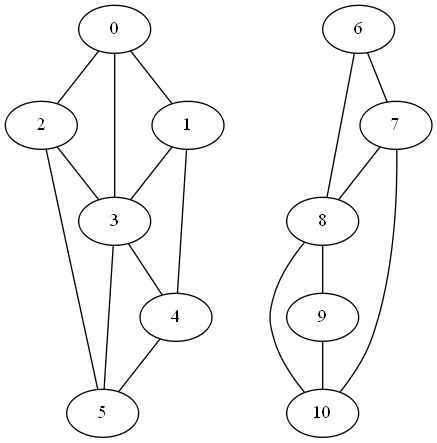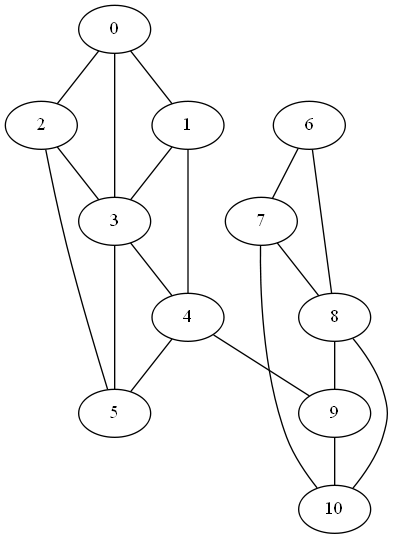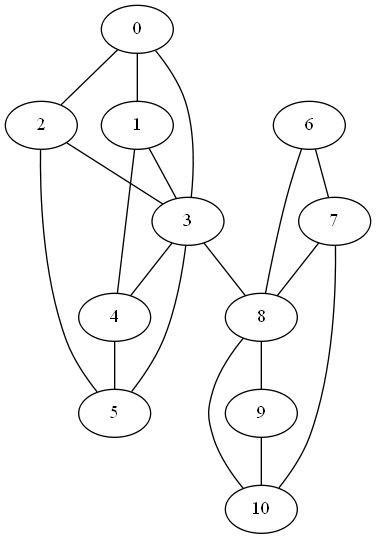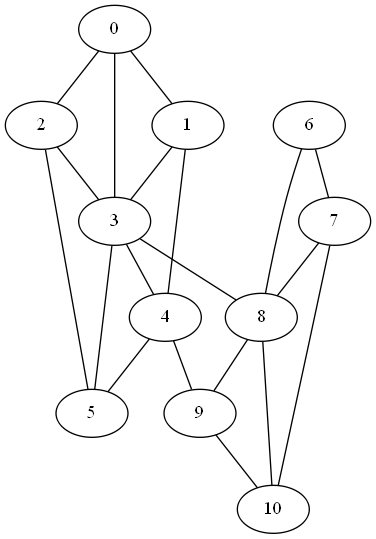# Measuring Connectivity with Graph Laplacian Eigenvalues

### Spectral graph theory, looking at the eigenvalues of the graph Laplacian, can tell us not just whether a graph is connected, but also how well it’s connected.

· Performance Zone · Analysis
Save
3.70K Views

if a graph can be split into two components with no links between them, that’s probably easy to see. it’s also unlikely unless there’s a good reason for it. the less obvious and more common case is a graph that can almost be split into two components.

the graph laplacian is the matrix l = d a where d is the diagonal matrix whose entries are the degrees of each node and a is the adjacency matrix. the smallest eigenvalue of l , λ 1 , is always 0. (why? see footnote .) the second smallest eigenvalue λ 2 tells you about the connectivity of the graph. if the graph has two disconnected components, λ 2 = 0. and if λ 2 is small , this suggests the graph is nearly disconnected, that it has two components that are not very connected to each other. in other words, the second eigenvalue gives you a sort of continuous measure of how well a graph is connected .

to illustrate this, we’ll start with a disconnected graph and see what happens to λ 2 as we add edges connecting its components.the first two eigenvalues of l are zero as expected. (actually, when i computed them numerically, i got values around 10 -15 , about 15 orders of magnitude smaller than the next eigenvalue, so the first two eigenvalues are zero to the limits of floating point precision.)

next, we add an edge between nodes 4 and 9 to form a weak link between the two clusters.in this graph, the second eigenvalue λ 2 jumps to 0.2144.

if we connect nodes 3 and 8 instead of 4 and 8, we create a stronger link between the components since nodes 3 and 8 have more connections in their respective components. now λ 2 becomes 0.2788.finally, if we add both, connecting nodes 4 and 9 and nodes 3 and 8, λ 2 increases to 0.4989.* * *

 to see why the smallest eigenvalue is always 0, note that v = (1, 1, 1, …, 1) is an eigenvector for 0. multiplying the i th row of d by v picks out the degree of node i . multiplying the i th row of a by v sums that row, which is also the degree of node i .

Topics:
connectivity, graph theory

Published at DZone with permission of John Cook, DZone MVB.

Opinions expressed by DZone contributors are their own.